# How to Find Maximum and Minimum Element in a Single Scan

## How to Find Maximum and Minimum Element in a Single Scan

In this article, I am going to discuss How to Find Maximum and Minimum Elements in an array in C Language in a Single Scan with Examples. In our previous article, where we discussed How to a Pair of Element with Sum K from a sorted Array in C Language with Examples

##### Find Maximum and Minimum Element from an Array in a Single Scan:

We have seen how to find maximum elements in our previous articles. But here we want to do this simultaneously in a single scan.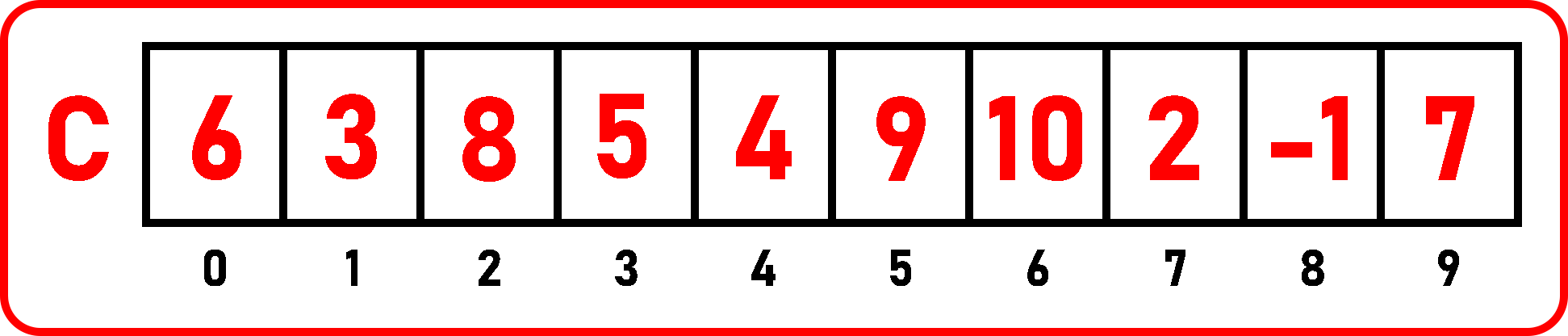We have taken an unsorted array of size 10. We have to find the max and min elements in this array. So let’s take two variables max and min to trace the array for maximum and minimum elements.

Let’s assign these two variables to C . So, max = 6, min = 6, Now take another variable ‘j’ at C :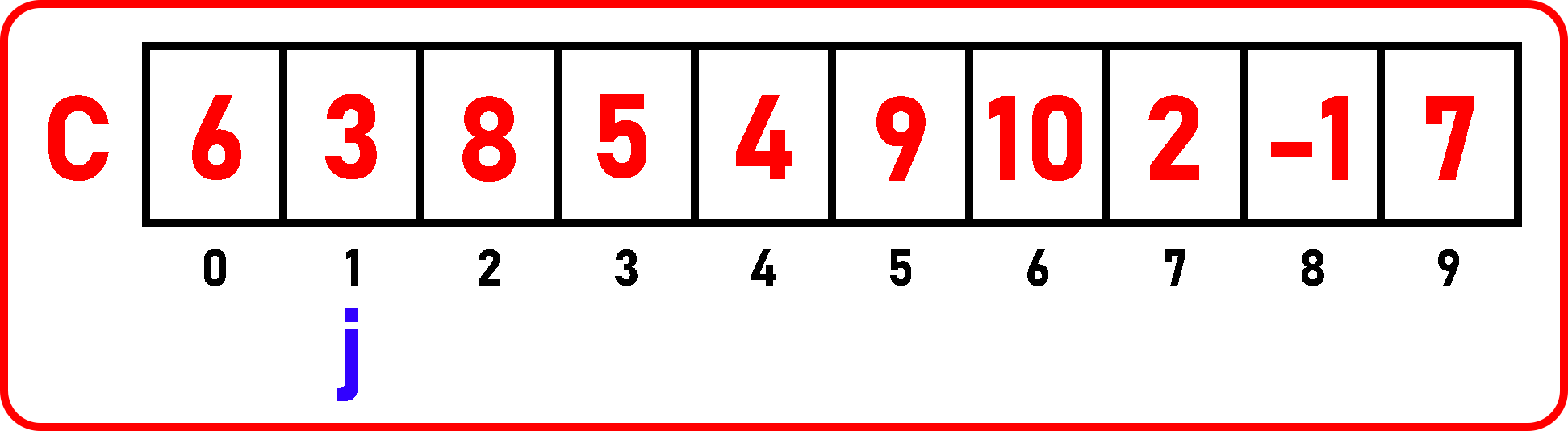Now j is pointing on 3. So, we will check here, If 3 < min? Yes, so modify the min variable and assign it to 3, 3 is less than min so, we don’t need to check for the max variable. So, variables are: max = 6, min = 3, Now move to the next element: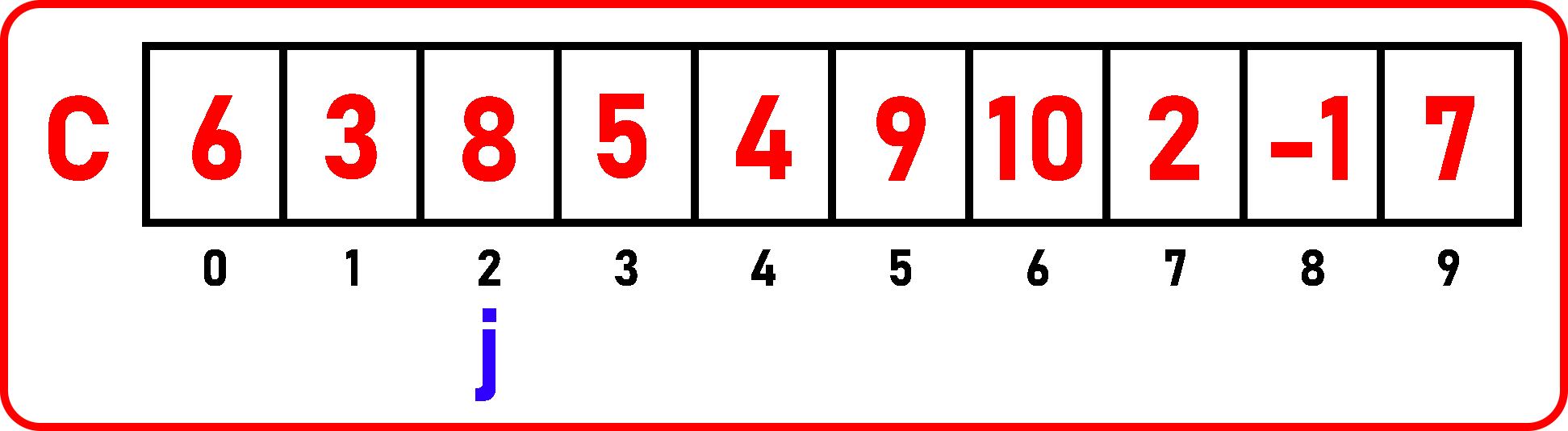Now j is pointing on 8. So, we will check here, If 8 < min? No, then check, If 8 > max? Yes, so modify the max variable and assign it to 8. Variables are: max = 8, min = 3, Now move to the next element: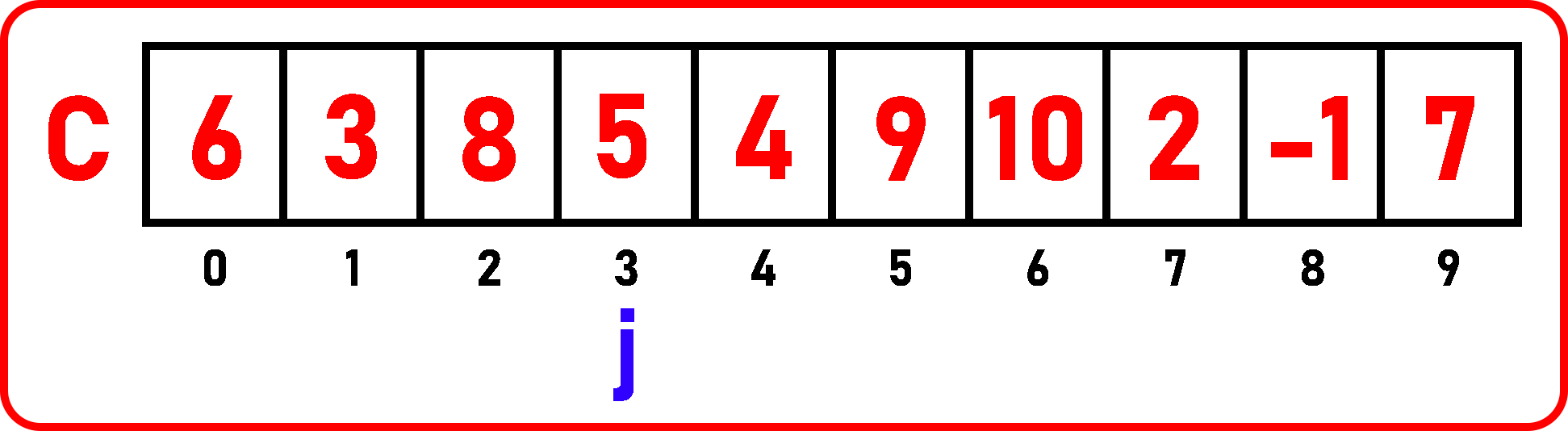Now j is pointing on 5. Variables are: max = 8, min = 3. So, we will check here, If 5 < min? No, then check, If 5 > max? No, so move to the next element: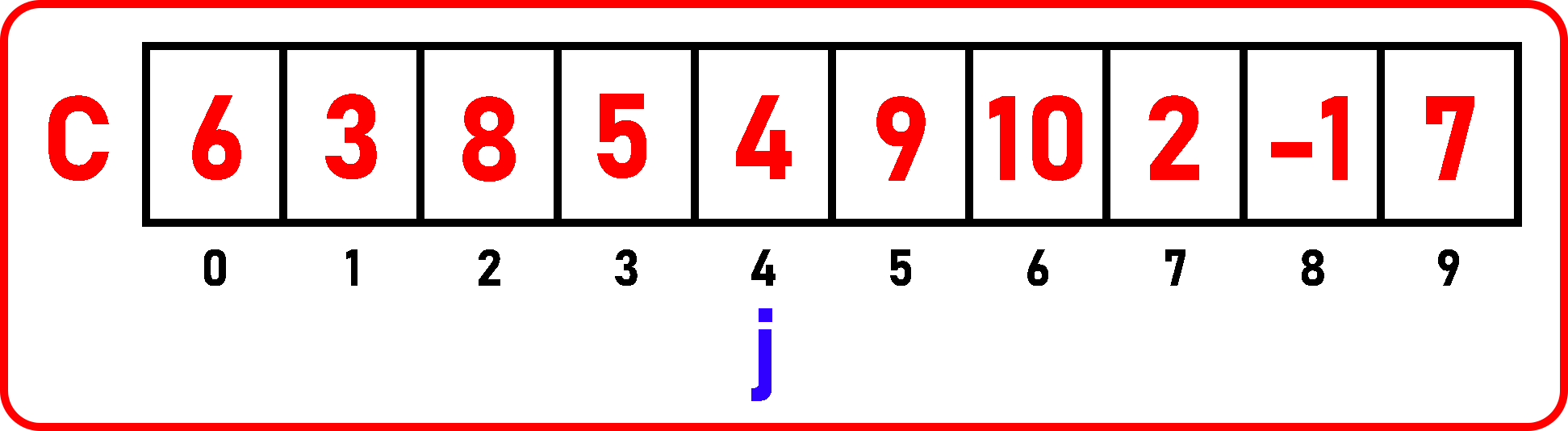Now j is pointing on 4. Variables are: max = 8, min = 3. So, we will check here, If 4 < min? No, then check, If 4 > max? No, so move to the next element: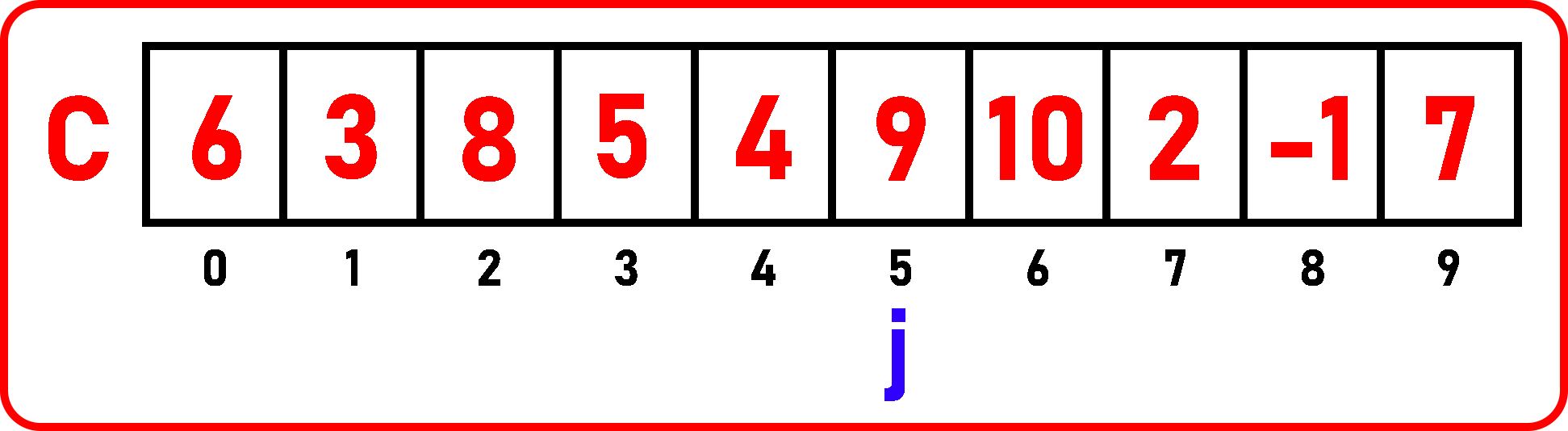Now j is pointing on 9. Variables are: max = 8, min = 3. So, we will check here, If 9 < min? No, then check, If 9 > max? Yes, so modify the max variable and assign it to 9. Variables are: max = 9, min = 3, Now move to the next element: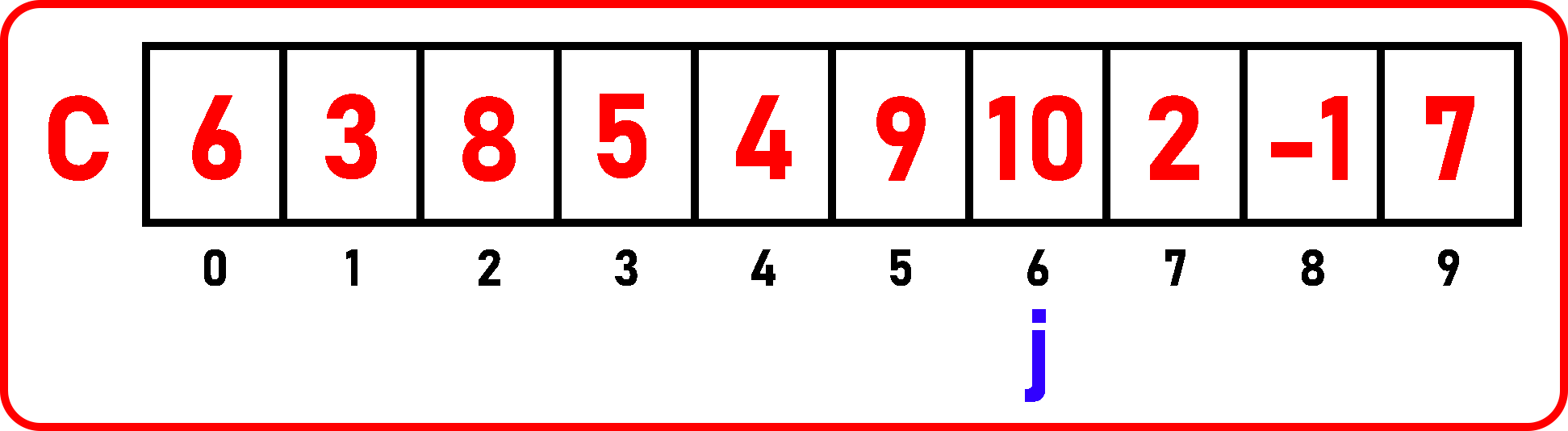Now j is pointing on 10. Variables are: max = 8, min = 3. So, we will check here, If 10 < min? No, then check, If 10 > max? Yes, so modify the max variable and assign it to 10. Variables are: max = 10, min = 3, Now move to the next element: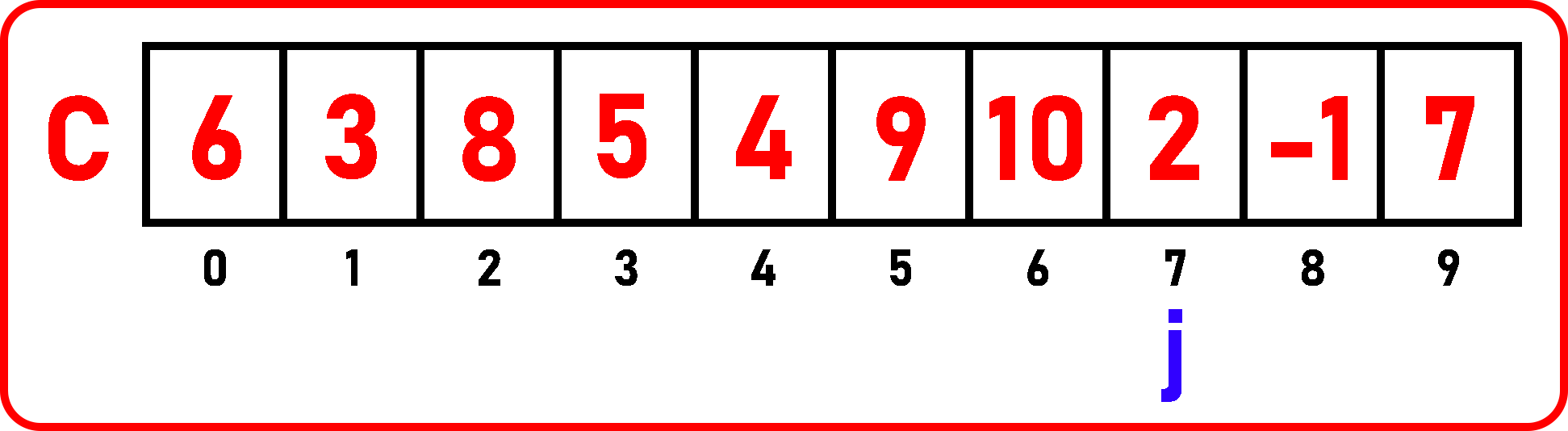Now j is pointing on 2. Variables are: max = 10, min = 3. So, we will check here, If 2 < min? Yes, so modify the min variable and assign it to 2, 2 is less than min so, we don’t need to check for the max variable. So, variables are: max = 10, min = 2, Now move to the next element: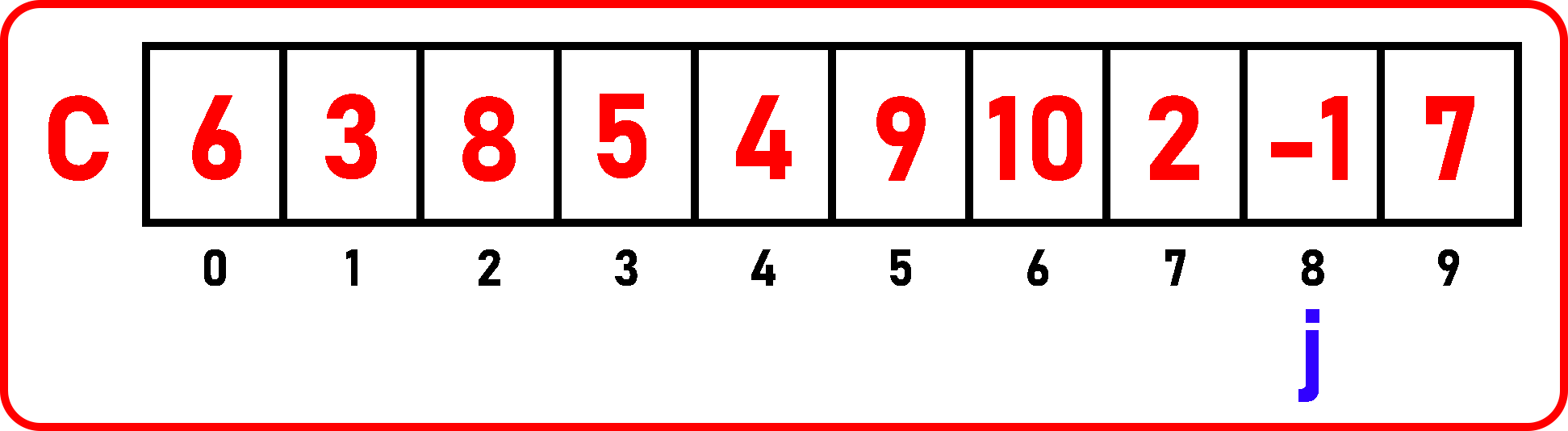Now j is pointing on -1. Variables are: max = 10, min = 2. So, we will check here, If -1 < min? Yes, so modify the min variable and assign it to -1, -1 is less than min so, we don’t need to check for the max variable. So, variables are: max = 10, min = -1, Now move to the next element: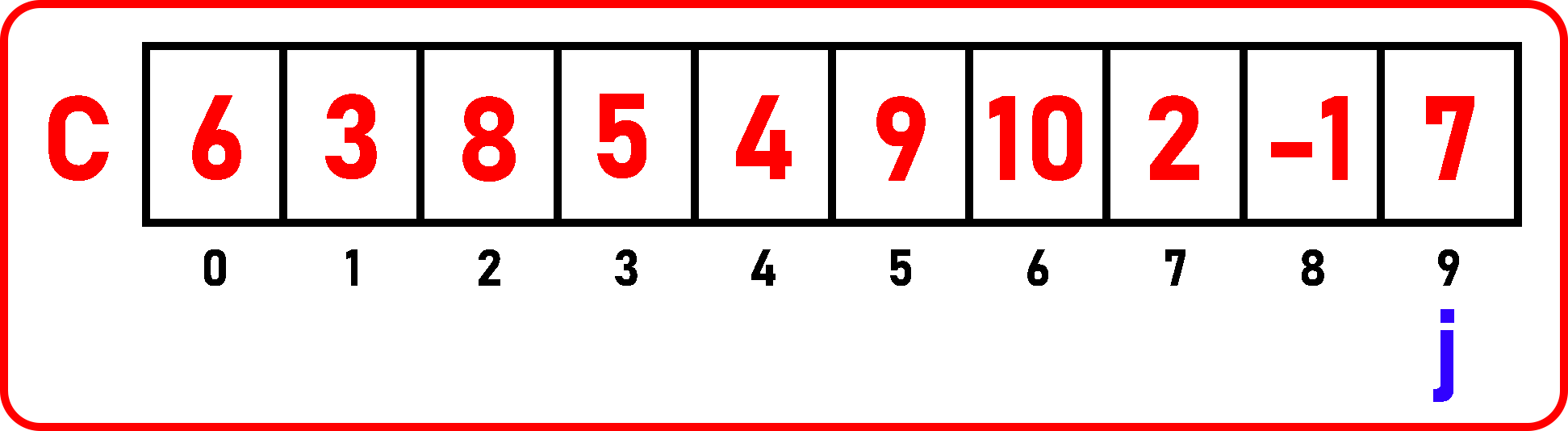Now j is pointing on 7. Variables are: max = 10, min = -1. So, we will check here,

If 7 < min? No, then check,

If 7 > max? No, so here we have traced the whole array and we get the maximum and minimum elements in a single scan: max = 10, min = -1.

Time Complexity: O (n)

1. Minimum Time: if the list is in descending order i.e. 10, 8, 6, 5, 4, 2… Here only one condition is executing which is for checking the min element in every iteration. So, Total No. of comparison: n-1 (Best Case)
2. Maximum Time: if the list is in ascending order i.e. 1, 2, 3, 4, 6, 8… Here both the conditions will execute in every iteration for checking min as well as max. So, Total No of comparisons: 2 * (n-1) (Worst Case)
##### Find Maximum and Minimum from an Array in a Single Scan Code in C Language:
```#include <stdio.h>
#include <stdlib.h>
struct List{
int C;
int size;
int length;
};

void Display(struct List list) {
int i;
printf("Elements are:\n");
for (i = 0;i<list.length;i++)
printf("%d ", list.C[i]);
printf("\n\n");
}

void MaxAndMin(struct List list){
int max = list.C, min = list.C;

for(int i = 1; i < list.length; i++){
if(list.C[i] < min){
min = list.C[i];
}
else if(list.C[i] > max){
max = list.C[i];
}
}
printf("Max Element: %d\nMin Element: %d", max, min);
}

int main(){
struct List list_1 = {{6, 3, 8, 5, 4, 9, 10, 2, -1, 7}, 10, 10};
Display(list_1);
MaxAndMin(list_1);
}

```
###### Output: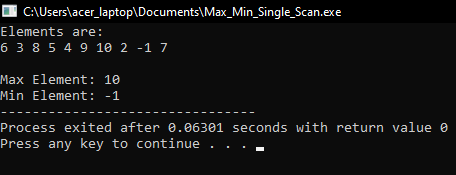In the next article, I am going to discuss Matrices with Examples. Here, in this article, I try to explain How to find maximum and minimum elements present in an array in C Language with Examples and I hope you enjoy this Finding maximum and minimum elements present in an array in C Language with Examples article.